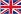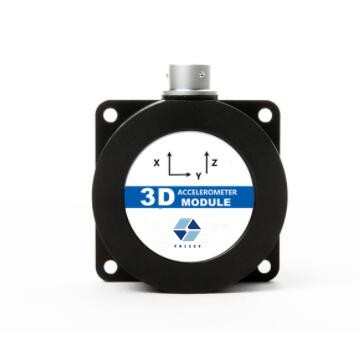Whats App : +8613992884879    WeChat : 13992884879    Email : info@ericcointernational.comEnglish
You are here: » » » What is the principle of inertial acceleration sensor?

# What is the principle of inertial acceleration sensor?

Views:2     Author:Site Editor     Publish Time: 2021-04-06      Origin:Site Inquire

At present, there is a large market demand for physical measurement of moving objects.Inertial acceleration sensor can be used to detect and detect the vibration, selection, acceleration of the object, it can efficiently solve the important components of navigation and carrier control, it makes full use of the inertia force of the sensor, to measure it.So what do you know about the inertial acceleration sensor?Next, by the sensor enthusiasts to take you to understand!What is the principle of inertial acceleration sensor ?

An inertial acceleration sensor usually includes an accelerometer, also known as an angular velocity sensor, or an acceleration sensor. Let's look at the inertial acceleration sensor principle.Inertial sensor generally includes accelerometer, it is also called acceleration sensor and angular velocity sensor, angular velocity sensor is also called gyroscope, here we mainly understand the basic principle of accelerometer and gyroscope.

Accelerometer is commonly by detection quality, bearing, potentiometer, springs, dampers, and housing, but at the same time is to use the basic principle of acceleration, counting objects in space motion state, namely the beginning accelerometer is induction surface vertical acceleration, is used in the early detection of aircraft overload instrument system.Then, through function upgrading and optimization, the main accelerometer that can recognize the acceleration of an object in any direction is the 3-axis accelerometer, which measures the acceleration data of an object on the three axes X, Y and Z in the spatial coordinate system, and can reflect the motion properties of the object's translation in all aspects.

How does an inertial sensor work?

Unlike accelerometer measurement dimensions, we according to the definition of gyroscope, understandable to the gyroscope is mainly by measuring the vertical axis of the gyro rotor in space coordinate system and the Angle between the object, now the mainstream of the gyroscope is three axis, the measuring objects on the X, Y, Z axis rotation, pitch, horizontal and vertical wave respectively.

The earliest gyroscopes are mechanical gyroscopes with high-speed rotating gyroscopes built in. Because gyroscopes can maintain high-speed and stable rotation on the universal support, the earliest gyroscopes are used to identify the direction, determine the attitude and calculate the angular velocity in navigation, and then are gradually applied to aircraft instruments.But the mechanical requirements for machining accuracy high, also very easy to influenced by external vibration, thus the calculation precision of mechanical gyroscope is always not tall.

What is the principle of inertial acceleration sensor?Inertial acceleration sensors, usually can be divided into two categories, one is angular rate gyro, and the other is line accelerometer.In industry, the principle of stable and efficient operation mechanism is widely used.Inertial acceleration sensor is different from the traditional acceleration sensor, and it is an important part to solve the problem of orientation, navigation and moving carrier control.

## CONTACT US

Whats App:+86-13992884879
 WeChat:13992884879

## SEND MESSAGE

Copyright © Ericco International Limited All rights reserved.  |  Support By Goodwaimao |  Sitemap